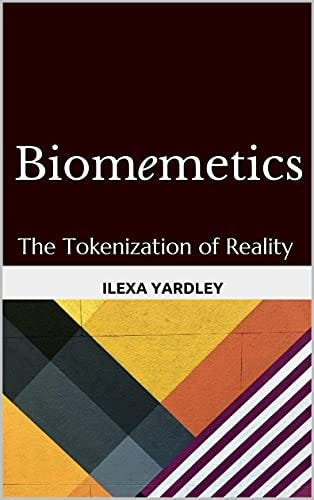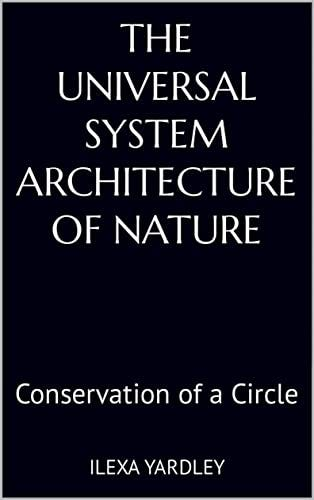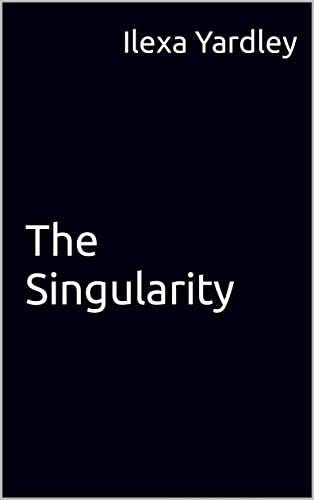# Quantum Reality

Quantum relativity: a singularity of singularities. One Zero One One (The tokenization of space.) (The ‘ matrix.’) (A metaverse.)

Quantum reality looks like this:

Thus, this:

This:

And, this:

And, also, this:

Any ‘matrix’ articulates the circular-linear (zero-one) relationship.

That is, the cell and its contents, like any X and X, articulate, and must conserve, a circle. No matter if the cell is blank or if it has ‘contents.’ Any combination of zeroes and ones (any number of cells) reduces and expands to one zero and one one (one circumference and one diameter, technically). Explaining one ‘cell’ and its circular-linear relationship to any number of ‘other’ cells. Explaining matrices, lattices, vortexes, edges, matrix mathematics, matrix manipulation, transformation, and, thus, everything in quantum reality (quantum physics) (reality at any ‘scale’).

Meaning, ‘pi’ is the correct technical term (mathematical term) for, what humans label , and experience as, ‘mind.’ (Mind and matter (X and X) articulate, and, thus, conserve, a circle.)

That is, any and all ‘observations’ of ‘reality’ (and, therefore, Nature) are the tokenization of a circle. Explaining everything in science, religion, and art. Life in general. (Finance in general.) (Tokenization in general.)

## Nature’s Algorithm

Thus, the program that controls reality is simple: if zero, then one. If one, then zero. Meaning, more technically, if circumference, then diameter. If diameter, then circumference.

This explains everything in Nature. And, everything in, what humans label, ‘reality.’ Also known as ‘absolute relativity.’ 50–50 as the ‘constant.’ And the norm. Giving us the architecture and the algorithm for ‘quantum relativity.’ (Gravity on all scales.)

## Universal Tokenization

Meaning, no matter what we do, as humans, we are mimicking everything in Nature, which is the tokenization of an uber-basic (and,thus, always conserved) circle. (Also known as biomemetics.)

Meaning, as we move from zero to one, we have automatically moved from one to zero (every movement makes the opposing movement ‘real.’) Explaining why humans (and all of the constituents of Nature) use 50–50 as the constant for everything. (If zero then one, if one then zero.)

## Spatial Topology

Why there is ‘space’ between any X and Y (X and X) (X and X’). What the correct tokenization of space looks like (the diagrams above and below). Why the diagrams in this article provide the correct topology for space. (For physicists and mathematicians and all the rest of us.)

And the only ‘operation’ in Nature.

Where, technically, then, we are ‘always’ tokenizing ‘space.’

## Self Referential Recursion

This is because you cannot have a half without a whole because, as tokenized above, you cannot have a circumference without a diameter.

Meaning, at all times half-of-me is X and half-of me is Y. Same for ‘you.’ And same for everything in Nature. Explaining human behavior (all of it) and Nature’s behavior (all of it). Magical thinking in general.

Half of me likes you. The other half, not at all. (Same for you.) Same for everything in Nature. At all times. In all places. In all relationships.

So, there it is.

## Conservation of the Circle: Core Dynamic in Nature

Conservation of a circle controls reality (your reality) (my reality). Nature’s reality. Easily mimicked with a computer. No matter the combination of zeroes and ones: one zero one one.

Why biomemetics explains reality. (Why all of the words in any language, including everything in mathematics and technology, are tokens for a circle.) (How all hash functions work.) (How all circuits work.) (How all ‘programs’ work.) (Why we ‘go to school’ to get ‘programmed’ (learn the hashtags) (tokens) for any discipline.)

Again, if zero then one, if one then zero, produces a perpetual machine (an infinite line of code) (reality in general) (life in general) (Nature in totality) (Nature as a singularity of singularities).Amazon.com: Biomemetics: The Tokenization of Reality eBook : Yardley, Ilexa: Kindle StoreAmazon.com: The Universal System Architecture of Nature: Conservation of a Circle eBook : Yardley, Ilexa: Kindle StoreThe Singularity , Yardley, Ilexa — Amazon.com

--

--